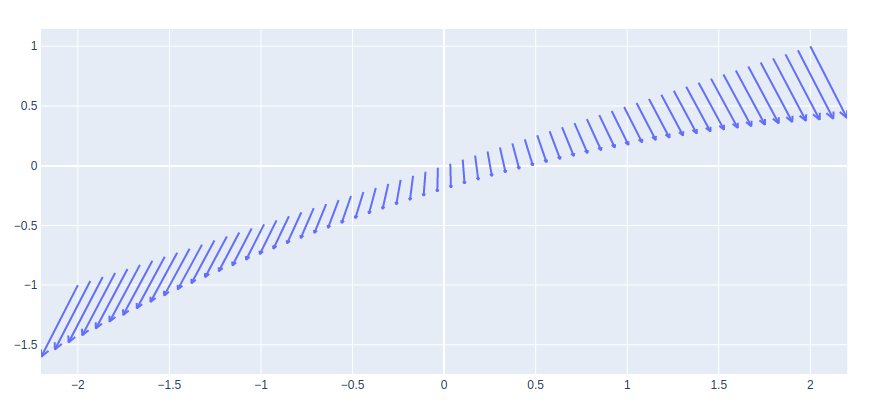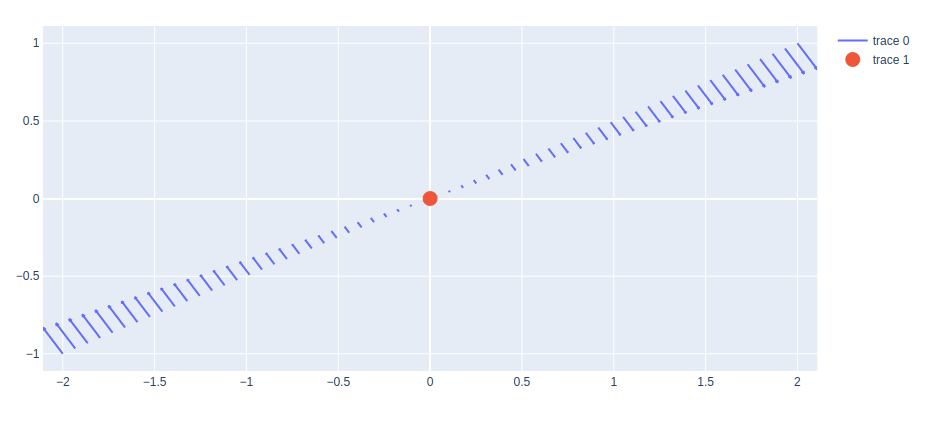Open in App
Not now

# Quiver Plots using Plotly in Python

• Last Updated : 05 Sep, 2020

A Plotly is a Python library that is used to design graphs, especially interactive graphs. It can plot various graphs and charts like histogram, barplot, boxplot, spreadplot, and many more. It is mainly used in data analysis as well as financial analysis. plotly is an interactive visualization library.

## Quiver Plots

A quiver plot displays velocity vectors as arrows with the components (u, v) at the points (x, y). The quiver(x, y, u, v) plots vectors as arrows at the coordinates which are specified in each corresponding pair of elements in x and y. The main advantage of using a quiver plot is it can represent a wider range of magnitudes without having the arrows shrink to dots or overlap one another.

Syntax: create_quiver(x, y, u, v, scale=0.1, arrow_scale=0.3, angle=0.3490658503988659, scaleratio=None)

Parameters:

x: x coordinates of the arrow locations

y: y coordinates of the arrow locations

u: x components of the arrow vectors

v: y components of the arrow vectors

arrow_scale: value multiplied to length of barb to get length of arrowhead. Default = .3

angle: angle of arrowhead. Default = pi/9

Example:

## Python3

 `import` `plotly.figure_factory as ff ` `import` `numpy as np ` ` `  `x ``=` `np.linspace(``-``2``, ``2``, ``60``) ` `y ``=` `np.linspace(``-``1``, ``1``, ``60``) ` `Y, X ``=` `np.meshgrid(x, y) ` `u ``=` `1` `-` `X``*``*``2` `+` `Y ` `v ``=` `-``1` `+` `X ``-` `Y``*``*``2` ` `  `# Create quiver plot ` `fig ``=` `ff.create_quiver(x, y, u, v, arrow_scale``=``.``1``) ` ` `  `fig.show()`

Output:## Plotting Points with Quiver Plot

A quiver plot can be shown with the points with the help of the add_trace() method of graph_objects class. The scatter plot is added in such a way that shows the origin of the quivers.

Example:

## Python3

 `import` `plotly.figure_factory as ff ` `import` `plotly.graph_objects as go ` `import` `numpy as np ` ` `  `x ``=` `np.linspace(``-``2``, ``2``, ``60``) ` `y ``=` `np.linspace(``-``1``, ``1``, ``60``) ` `Y, X ``=` `np.meshgrid(x, y) ` `u ``=` `np.cos(X)``*``Y ` `v ``=` `np.sin(X)``*``Y ` ` `  `# Create quiver plot ` `fig ``=` `ff.create_quiver(x, y, u, v, arrow_scale``=``.``1``) ` ` `  `# Adding scatter as the origin ` `fig.add_trace(go.Scatter(x ``=` `[``0``], y ``=` `[``0``], ` `                        ``mode ``=` `'markers'``, ` `                         ``marker_size ``=` `15` `                        ``)) ` ` `  `fig.show()`

Output:My Personal Notes arrow_drop_up
Related Articles